# Classification du Raclette à la Tensor¶

Raclette is a dish indigenous to parts of Switzerland. The raclette cheese round is heated, either in front of a fire or by a special machine, then scraped onto diners' plates; the term raclette derives from the French word racler, meaning "to scrape", a reference to the fact that the melted cheese must be scraped from the unmelted part of the cheese onto the plate. (Wikipedia)

This notebook created in clear imitation of Not Hotdog at the Open Food Data Hackdays / Applied Machine Learning Days, and was based on code and tips from:

..the underlying model used is ResNet-18, described here:

..and this was also motivated by this tweet:

For an introduction to PyTorch and Tensors, start here (pytorch.org)

To skip right to the juicy part, click here.

In :
import torch
import torch.nn as nn
from torch.autograd import Variable
import torchvision
from torch.utils.data import DataLoader
from torchvision import transforms
from torchvision.datasets import ImageFolder
import matplotlib.pyplot as plt
from PIL import Image


Experiencing a problem here? You probably need to conda install or pip install some more lovely packages.

In :
data_dir = "data"


Create a data folder with subfolders, each containing JPG images, e.g.:

data/raclette/image_1.jpg
data/not_raclette/image_2.jpg

The subfolder name (e.g. raclette) will correspond to our label. You can pick test images from the folder here.

Here the main set up work takes place, depending on how many images you have ImageFolder may take a while:

In :
# dataset pre-processing
train_transform = transforms.Compose([
transforms.CenterCrop(200), # crop our images to a 200 square
transforms.Resize(224), # resize down to 224x224 (power of 2)
transforms.ToTensor(), # convert to a Tensor
])

# details on how to use ImageFolder in https://github.com/pytorch/vision#imagefolder
tset = ImageFolder(data_dir, transform=train_transform)
train_dataloader = DataLoader(tset, batch_size=4, shuffle=True)
num_classes = len(set(tset.classes))

# use pretrained resnet18 network with image input
model = torchvision.models.resnet18(pretrained=True)
num_ftrs = model.fc.in_features # e.g. 2048
model.fc = torch.nn.Linear(num_ftrs, num_classes)
print("Features: ", num_ftrs)

# run in cuda
if torch.cuda.is_available():
model = model.cuda()
print(model)

Features:  512
ResNet (
(conv1): Conv2d(3, 64, kernel_size=(7, 7), stride=(2, 2), padding=(3, 3), bias=False)
(bn1): BatchNorm2d(64, eps=1e-05, momentum=0.1, affine=True)
(relu): ReLU (inplace)
(maxpool): MaxPool2d (size=(3, 3), stride=(2, 2), padding=(1, 1), dilation=(1, 1))
(layer1): Sequential (
(0): BasicBlock (
(conv1): Conv2d(64, 64, kernel_size=(3, 3), stride=(1, 1), padding=(1, 1), bias=False)
(bn1): BatchNorm2d(64, eps=1e-05, momentum=0.1, affine=True)
(relu): ReLU (inplace)
(conv2): Conv2d(64, 64, kernel_size=(3, 3), stride=(1, 1), padding=(1, 1), bias=False)
(bn2): BatchNorm2d(64, eps=1e-05, momentum=0.1, affine=True)
)
(1): BasicBlock (
(conv1): Conv2d(64, 64, kernel_size=(3, 3), stride=(1, 1), padding=(1, 1), bias=False)
(bn1): BatchNorm2d(64, eps=1e-05, momentum=0.1, affine=True)
(relu): ReLU (inplace)
(conv2): Conv2d(64, 64, kernel_size=(3, 3), stride=(1, 1), padding=(1, 1), bias=False)
(bn2): BatchNorm2d(64, eps=1e-05, momentum=0.1, affine=True)
)
)
(layer2): Sequential (
(0): BasicBlock (
(conv1): Conv2d(64, 128, kernel_size=(3, 3), stride=(2, 2), padding=(1, 1), bias=False)
(bn1): BatchNorm2d(128, eps=1e-05, momentum=0.1, affine=True)
(relu): ReLU (inplace)
(conv2): Conv2d(128, 128, kernel_size=(3, 3), stride=(1, 1), padding=(1, 1), bias=False)
(bn2): BatchNorm2d(128, eps=1e-05, momentum=0.1, affine=True)
(downsample): Sequential (
(0): Conv2d(64, 128, kernel_size=(1, 1), stride=(2, 2), bias=False)
(1): BatchNorm2d(128, eps=1e-05, momentum=0.1, affine=True)
)
)
(1): BasicBlock (
(conv1): Conv2d(128, 128, kernel_size=(3, 3), stride=(1, 1), padding=(1, 1), bias=False)
(bn1): BatchNorm2d(128, eps=1e-05, momentum=0.1, affine=True)
(relu): ReLU (inplace)
(conv2): Conv2d(128, 128, kernel_size=(3, 3), stride=(1, 1), padding=(1, 1), bias=False)
(bn2): BatchNorm2d(128, eps=1e-05, momentum=0.1, affine=True)
)
)
(layer3): Sequential (
(0): BasicBlock (
(conv1): Conv2d(128, 256, kernel_size=(3, 3), stride=(2, 2), padding=(1, 1), bias=False)
(bn1): BatchNorm2d(256, eps=1e-05, momentum=0.1, affine=True)
(relu): ReLU (inplace)
(conv2): Conv2d(256, 256, kernel_size=(3, 3), stride=(1, 1), padding=(1, 1), bias=False)
(bn2): BatchNorm2d(256, eps=1e-05, momentum=0.1, affine=True)
(downsample): Sequential (
(0): Conv2d(128, 256, kernel_size=(1, 1), stride=(2, 2), bias=False)
(1): BatchNorm2d(256, eps=1e-05, momentum=0.1, affine=True)
)
)
(1): BasicBlock (
(conv1): Conv2d(256, 256, kernel_size=(3, 3), stride=(1, 1), padding=(1, 1), bias=False)
(bn1): BatchNorm2d(256, eps=1e-05, momentum=0.1, affine=True)
(relu): ReLU (inplace)
(conv2): Conv2d(256, 256, kernel_size=(3, 3), stride=(1, 1), padding=(1, 1), bias=False)
(bn2): BatchNorm2d(256, eps=1e-05, momentum=0.1, affine=True)
)
)
(layer4): Sequential (
(0): BasicBlock (
(conv1): Conv2d(256, 512, kernel_size=(3, 3), stride=(2, 2), padding=(1, 1), bias=False)
(bn1): BatchNorm2d(512, eps=1e-05, momentum=0.1, affine=True)
(relu): ReLU (inplace)
(conv2): Conv2d(512, 512, kernel_size=(3, 3), stride=(1, 1), padding=(1, 1), bias=False)
(bn2): BatchNorm2d(512, eps=1e-05, momentum=0.1, affine=True)
(downsample): Sequential (
(0): Conv2d(256, 512, kernel_size=(1, 1), stride=(2, 2), bias=False)
(1): BatchNorm2d(512, eps=1e-05, momentum=0.1, affine=True)
)
)
(1): BasicBlock (
(conv1): Conv2d(512, 512, kernel_size=(3, 3), stride=(1, 1), padding=(1, 1), bias=False)
(bn1): BatchNorm2d(512, eps=1e-05, momentum=0.1, affine=True)
(relu): ReLU (inplace)
(conv2): Conv2d(512, 512, kernel_size=(3, 3), stride=(1, 1), padding=(1, 1), bias=False)
(bn2): BatchNorm2d(512, eps=1e-05, momentum=0.1, affine=True)
)
)
(avgpool): AvgPool2d (
)
(fc): Linear (512 -> 2)
)


You may ponder the runes above for a while, which explain the model that was just constructed.

Now we are ready to load the data.

In :
# optimizer
optimizer = torch.optim.SGD(model.parameters(), lr=0.001, momentum=0.9)

# loss function
loss_fn = nn.CrossEntropyLoss()

# begin to train models
model.train()

# getting an error here? install the latest torchvision
# pip install https://github.com/pytorch/vision/archive/master.zip
for inputs, labels in train_dataloader:
if torch.cuda.is_available():
# use cuda
inputs = Variable(inputs.cuda())
labels = Variable(labels.cuda())
else:
# use cpu
inputs = Variable(inputs)
labels = Variable(labels)

# zero the parameter gradients
optimizer.zero_grad()

# forward
outputs = model(inputs)
loss = loss_fn(outputs, labels)
loss.backward()
optimizer.step()


At this point it may be wise to go and brew yourself a hot cup of herbal tea from the Swiss alps.

In :
model.eval()

Out:
ResNet (
(conv1): Conv2d(3, 64, kernel_size=(7, 7), stride=(2, 2), padding=(3, 3), bias=False)
(bn1): BatchNorm2d(64, eps=1e-05, momentum=0.1, affine=True)
(relu): ReLU (inplace)
(maxpool): MaxPool2d (size=(3, 3), stride=(2, 2), padding=(1, 1), dilation=(1, 1))
(layer1): Sequential (
(0): BasicBlock (
(conv1): Conv2d(64, 64, kernel_size=(3, 3), stride=(1, 1), padding=(1, 1), bias=False)
(bn1): BatchNorm2d(64, eps=1e-05, momentum=0.1, affine=True)
(relu): ReLU (inplace)
(conv2): Conv2d(64, 64, kernel_size=(3, 3), stride=(1, 1), padding=(1, 1), bias=False)
(bn2): BatchNorm2d(64, eps=1e-05, momentum=0.1, affine=True)
)
(1): BasicBlock (
(conv1): Conv2d(64, 64, kernel_size=(3, 3), stride=(1, 1), padding=(1, 1), bias=False)
(bn1): BatchNorm2d(64, eps=1e-05, momentum=0.1, affine=True)
(relu): ReLU (inplace)
(conv2): Conv2d(64, 64, kernel_size=(3, 3), stride=(1, 1), padding=(1, 1), bias=False)
(bn2): BatchNorm2d(64, eps=1e-05, momentum=0.1, affine=True)
)
)
(layer2): Sequential (
(0): BasicBlock (
(conv1): Conv2d(64, 128, kernel_size=(3, 3), stride=(2, 2), padding=(1, 1), bias=False)
(bn1): BatchNorm2d(128, eps=1e-05, momentum=0.1, affine=True)
(relu): ReLU (inplace)
(conv2): Conv2d(128, 128, kernel_size=(3, 3), stride=(1, 1), padding=(1, 1), bias=False)
(bn2): BatchNorm2d(128, eps=1e-05, momentum=0.1, affine=True)
(downsample): Sequential (
(0): Conv2d(64, 128, kernel_size=(1, 1), stride=(2, 2), bias=False)
(1): BatchNorm2d(128, eps=1e-05, momentum=0.1, affine=True)
)
)
(1): BasicBlock (
(conv1): Conv2d(128, 128, kernel_size=(3, 3), stride=(1, 1), padding=(1, 1), bias=False)
(bn1): BatchNorm2d(128, eps=1e-05, momentum=0.1, affine=True)
(relu): ReLU (inplace)
(conv2): Conv2d(128, 128, kernel_size=(3, 3), stride=(1, 1), padding=(1, 1), bias=False)
(bn2): BatchNorm2d(128, eps=1e-05, momentum=0.1, affine=True)
)
)
(layer3): Sequential (
(0): BasicBlock (
(conv1): Conv2d(128, 256, kernel_size=(3, 3), stride=(2, 2), padding=(1, 1), bias=False)
(bn1): BatchNorm2d(256, eps=1e-05, momentum=0.1, affine=True)
(relu): ReLU (inplace)
(conv2): Conv2d(256, 256, kernel_size=(3, 3), stride=(1, 1), padding=(1, 1), bias=False)
(bn2): BatchNorm2d(256, eps=1e-05, momentum=0.1, affine=True)
(downsample): Sequential (
(0): Conv2d(128, 256, kernel_size=(1, 1), stride=(2, 2), bias=False)
(1): BatchNorm2d(256, eps=1e-05, momentum=0.1, affine=True)
)
)
(1): BasicBlock (
(conv1): Conv2d(256, 256, kernel_size=(3, 3), stride=(1, 1), padding=(1, 1), bias=False)
(bn1): BatchNorm2d(256, eps=1e-05, momentum=0.1, affine=True)
(relu): ReLU (inplace)
(conv2): Conv2d(256, 256, kernel_size=(3, 3), stride=(1, 1), padding=(1, 1), bias=False)
(bn2): BatchNorm2d(256, eps=1e-05, momentum=0.1, affine=True)
)
)
(layer4): Sequential (
(0): BasicBlock (
(conv1): Conv2d(256, 512, kernel_size=(3, 3), stride=(2, 2), padding=(1, 1), bias=False)
(bn1): BatchNorm2d(512, eps=1e-05, momentum=0.1, affine=True)
(relu): ReLU (inplace)
(conv2): Conv2d(512, 512, kernel_size=(3, 3), stride=(1, 1), padding=(1, 1), bias=False)
(bn2): BatchNorm2d(512, eps=1e-05, momentum=0.1, affine=True)
(downsample): Sequential (
(0): Conv2d(256, 512, kernel_size=(1, 1), stride=(2, 2), bias=False)
(1): BatchNorm2d(512, eps=1e-05, momentum=0.1, affine=True)
)
)
(1): BasicBlock (
(conv1): Conv2d(512, 512, kernel_size=(3, 3), stride=(1, 1), padding=(1, 1), bias=False)
(bn1): BatchNorm2d(512, eps=1e-05, momentum=0.1, affine=True)
(relu): ReLU (inplace)
(conv2): Conv2d(512, 512, kernel_size=(3, 3), stride=(1, 1), padding=(1, 1), bias=False)
(bn2): BatchNorm2d(512, eps=1e-05, momentum=0.1, affine=True)
)
)
(avgpool): AvgPool2d (
)
(fc): Linear (512 -> 2)
)
In :
def test_image(image_file):
plt.imshow(plt.imread(image_file))

img2 = Image.open(image_file)
img2 = train_transform(img2)
img2 = img2.unsqueeze(0)  # pytorch only accepts batch images

img2 = Variable(img2)
if torch.cuda.is_available(): img2 = img2.cuda()

target = model(img2)

# get the predict classes
_, pred = torch.max(target.data, 1)

print(nn.functional.softmax(target))
print("It must be: ", tset.classes[pred], " !!!")


## Testing¶

With a handy function we can throw a bunch of images (from outside of the training set) at the classifier, and see what happens.

In :
test_image("data/test/r1.jpg")

Variable containing:
0.4582  0.5418
[torch.FloatTensor of size 1x2]

It must be:  raclette  !!!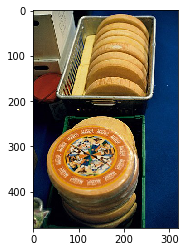Source: Raclette-Negative CC BY-SA 2.0 France Rama

In :
test_image("data/test/n1.jpg")

Variable containing:
0.8575  0.1425
[torch.FloatTensor of size 1x2]

It must be:  not_raclette  !!!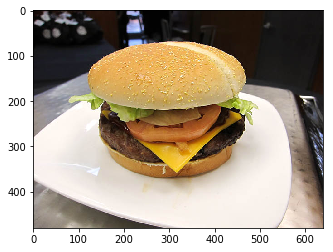In :
test_image("data/test/r2.jpg")

Variable containing:
0.0843  0.9157
[torch.FloatTensor of size 1x2]

It must be:  raclette  !!!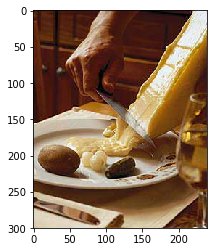Source: Raclette2 CC BY-SA 3.0 Grcampbell

In :
test_image("data/test/n2.jpg")

Variable containing:
0.9848  0.0152
[torch.FloatTensor of size 1x2]

It must be:  not_raclette  !!!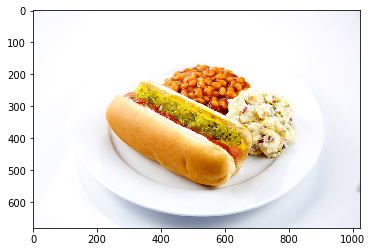In :
test_image("data/test/r3.jpg")

Variable containing:
0.4658  0.5342
[torch.FloatTensor of size 1x2]

It must be:  raclette  !!!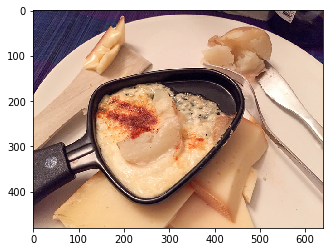Source: Christmas Raclette CC BY-SA 2.0 Kent Wang

In :
test_image("data/test/n3.jpg")

Variable containing:
0.9081  0.0919
[torch.FloatTensor of size 1x2]

It must be:  not_raclette  !!!